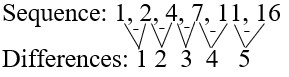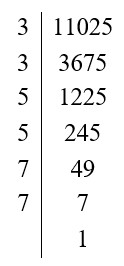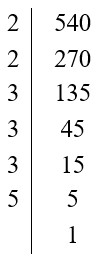4 Ijesha Close, Ilupeju, Lagos
+2348 097 685 118

#### Number Patterns and Sequences• Patterns occur when a sequence of numbers obeys a certain rule. For example:
• 1, 3, 6, 10, 15, … are triangular numbers.
• 1, 4, 9, 16, 25, … are square numbers.
• 1, 1, 2, 3, 5, 8, 13, … is a Fibonacci sequence.
• 3, 6, 9, 12, 15, … are multiples of 3.
• Number patterns can be shown in a diagram or in a simple graph.
• If a × a = b, then b is the square of a, and a is the square root of b. For example:
• Since 4 × 4 = 16, 16 is the square of 4, and 4 is the square root of 16.
• If the square root of a number, p, is a whole number, then p is a perfect square. For example:
• 121 is a perfect square because its square root is 11 (a whole number).
###### Examples
1. Find the next four terms in the sequence 1, 2, 4, 7, 11, 16, … (Method: Find the difference between two consecutive numbers)
•• Notice the pattern in the differences. The differences increase by 1 each time.
• The next term in the sequence is found by adding 6 to 16, which gives 22.
• The next term is found by adding 7 to 22, and so on.
• ∴ The next four terms are: 22, 29, 37, and 46.
2. Find √11025. (Hint: Try the prime numbers 2, 3, 5 ,7, … in turn)
• Working:• 11025 = 3² × 5² × 7²
•           = (3 × 5 × 7) × (3 × 5 × 7)
•           = 105 × 105
• ∴ √11025 = 105
• NOTE: It is not necessary to write a number in its prime factors.
3. Find √6400
• 6400 = 64 × 100
•          = 8² × 10²
• Thus = √6400 = 8 × 10 = 80
4. Find the smallest number which 540 must be multiplied so that the product is a perfect square.• 540 = 2² × 3² × 5
• The index of 2 is even.
• The indices of 3, and 5 are odd.
• One more 3 and one more 5 will make all the indices even. the product will then be a perfect square.
• The number required = 3 × 5 = 15
• What are the next two values in the following sequence?
1, 8, 27, 64, 125, 216, …

• If you check, the first term is the cube of 1; 1³ = 1.
• The second term is the cube of 2; 2³ = 8.
• The third term is the cube of 3; 3³ = 27.
• ∴ The seventh term would be 7³ = 343.
• ∴ The eighth term would be 8³ = 512
• What are the next two values in the following sequence?
15, 17, 20, 24, 29, 35, …

• The difference between the first and second term is 2; 17 – 15 = 2.
• The difference between the third and second term is 3; 20 – 17 = 3.
• The difference between the fourth and third term is 4; 24 – 20 = 4.
• ∴ The difference between the seventh and sixth term has to be 7:
• ∴ x – 35 = 7
• ∴ x = 42
• ∴ The seventh term is 42.
• ∴ The difference between the eighth and seventh term has to be 8:
• ∴ y – 42 = 8
• ∴ y = 50
• ∴ The eighth term is 50.